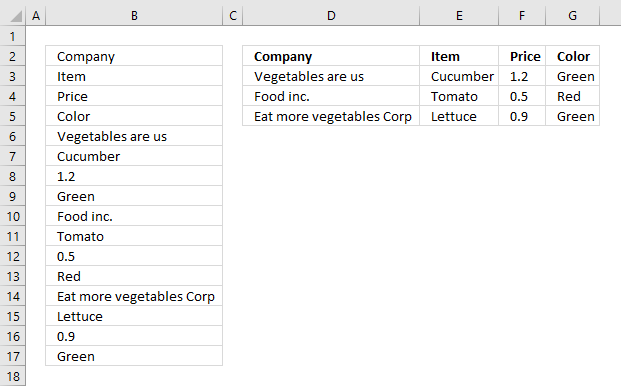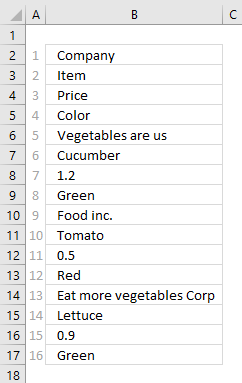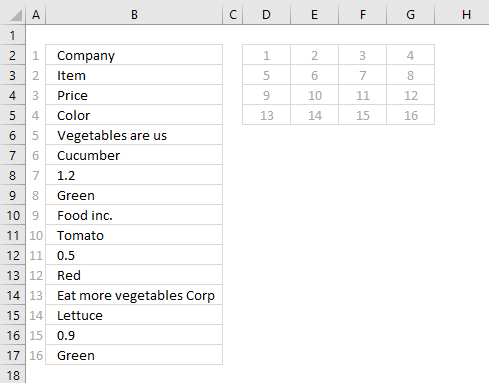Author: Oscar Cronquist Article last updated on November 15, 2018The picture above shows data presented in only one column (column B), this happens sometimes when you get an undesired output from a database or copying values from an HTML file.

The INDEX function allows you to easily rearrange values on an Excel worksheet, in this case, data seems to be grouped record by record. 4 values in one record.

Column D to G shows you the INDEX function rearranging the data.

### How to build the formula

The INDEX function has three arguments: INDEX(reference, row_num, [column_num])

The reference in the first argument points to cell range B2:B17. To return a value from that cell range you must know where it is, the INDEX function uses a row and column number to locate a particular value. Since this cell range (B2:B17) only has one column you only need to use a row number to get the value you want.The picture above has relative row numbers in column A to show you what number the INDEX function needs for it to return a specific value from cell range B2:B17. The values in column B seems to repeat, every 4th row has a company name. We can use that information to build the formula that returns a record in a row each.

The formula needs numbers in a determined sequence depending where on the worksheet it is. If I enter the formula in cell D2 it must get values in the order shown in the picture below.Combining the ROWS function and the COLUMNS function lets you build the number sequence shown above.

Formula in cell D2:

=INDEX(\$B\$2:\$B\$17,COLUMNS(\$A\$1:A1)+ROWS(\$A\$1:A1)*4-4)

COLUMNS(\$A\$1:A1) returns 1 and ROWS(\$A\$1:A1)*4-4 returns 0 (zero). 1 +0 is 1. The INDEX function returns the first value in cell range B2:B17 which is "Company".

In cell E2 COLUMNS(\$A\$1:A2) returns 2 and ROWS(\$A\$1:A1)*4-4 returns 0 (zero). 2+0 is 2. The INDEX function returns the second value in cell range B2:B17 which is "Item".

In cell D3 COLUMNS(\$A\$1:A1) returns 1 and ROWS(\$A\$1:A2)*4-4 returns 4. 1+4 is 5. The INDEX function returns the fifth value in cell range B2:B17 which is "Vegetables are us".